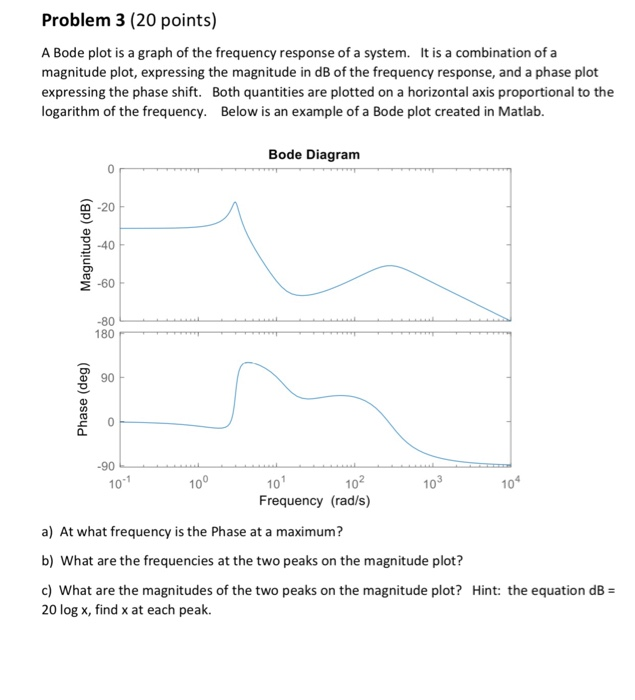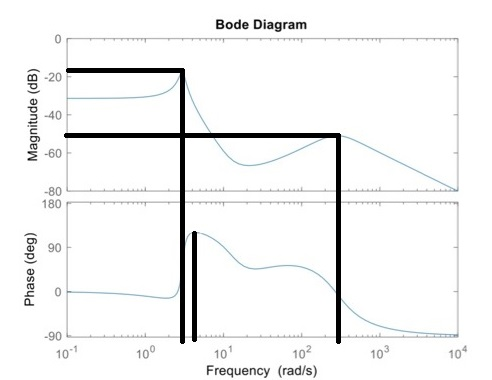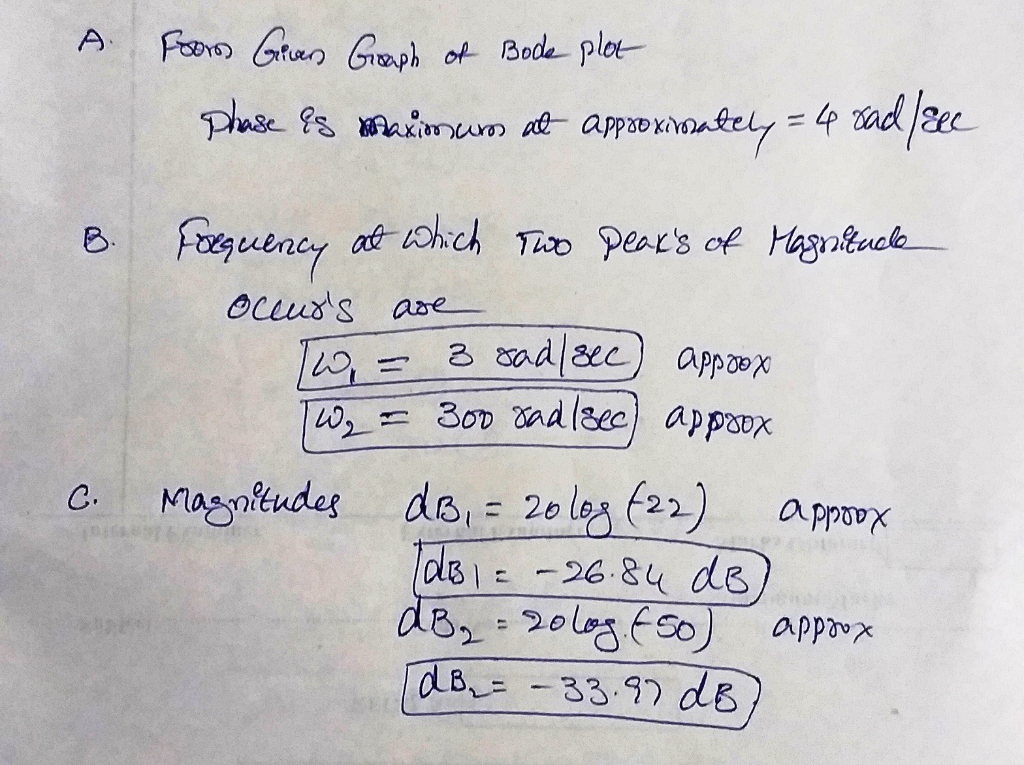# Problem 3 (20 points) A Bode plot is a graph of the frequency response of a system. It is a combi...Problem 3 (20 points) A Bode plot is a graph of the frequency response of a system. It is a combination of a magnitude plot, expressing the magnitude in dB of the frequency response, and a phase plot expressing the phase shift. Both quantities are plotted on a horizontal axis proportional to the logarithm of the frequency. Below is an example of a Bode plot created in Matlab. Bode Diagram 0 -20 3-40 E-60 -80 180 90 10 10 10 Frequency (rad/s) 10 10 10 a) At what frequency is the Phase at a maximum? b) What are the frequencies at the two peaks on the magnitude plot? c) What are the magnitudes of the two peaks on the magnitude plot? Hint: the equation dB 20 log x, find x at each peak.##### Add Answer of: Problem 3 (20 points) A Bode plot is a graph of the frequency response of a system. It is a combi...
More Homework Help Questions Additional questions in this topic.

• #### Bode Plot Problem

Need Online Homework Help?